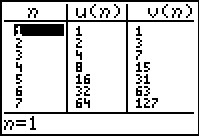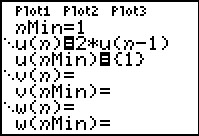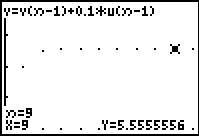•• ##### Device
•TI-84 Plus CE
• TI-84 Plus
• TI-83 Plus Family

# SA and NT: Geometric Sequences & Series

by Texas Instruments#### Overview

Students find common ratios of geometric sequences on a spreadsheet and create scatter plots of the sequences to see how each curve is related to the value of the common ratio and/or the sign of the first term of the sequence.

#### Key Steps

•Students will use the Sequence mode of the graphing calculator to generate the terms of a sequence. They view the table of values to determine any patterns, rate of change, and function that could produce the same table.

•Then, students will generate the series or the partial sums of the sequence. They will determine the relationship between the sequence and the series and see if the series converges or diverges.

•After investigating the table of values for a sequence and series that converges, students will view the graph of the series to determine what happens as n increases.